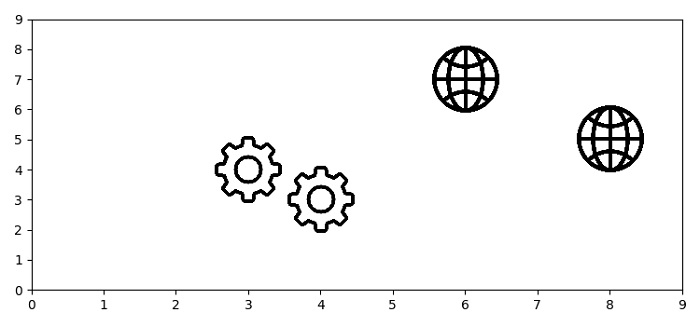# How to use a custom png image marker in a plot (Matplotlib)?

To use custome png or jpg i.e an image as a marker in a plot, we can take the following steps −

• Set the figure size and adjust the padding between and around the subplots.

• Make a paths list to store the directories of images.

• Make a list (x and y) of points.

• Using subplots() method, create a figure and a set of subplots.

• To plot images instead of points, iterate zipped x, y and paths.

• Instantiate AnnotationBbox() with image and (x, y) points.

• Put xticks and yticks on both the axes.

• To display the figure, use show() method.

## Example

import matplotlib.pyplot as plt
from matplotlib.offsetbox import OffsetImage, AnnotationBbox

plt.rcParams["figure.figsize"] = [7.50, 3.50]
plt.rcParams["figure.autolayout"] = True

def getImage(path):

paths = ['globe.jpg', 'settings.jpg', 'settings.jpg', 'globe.jpg']
x = [8, 4, 3, 6]
y = [5, 3, 4, 7]
fig, ax = plt.subplots()
for x0, y0, path in zip(x, y, paths):
ab = AnnotationBbox(getImage(path), (x0, y0), frameon=False)
plt.show()